Constructing line parallel to given line

Practical Geometry
Serial order wise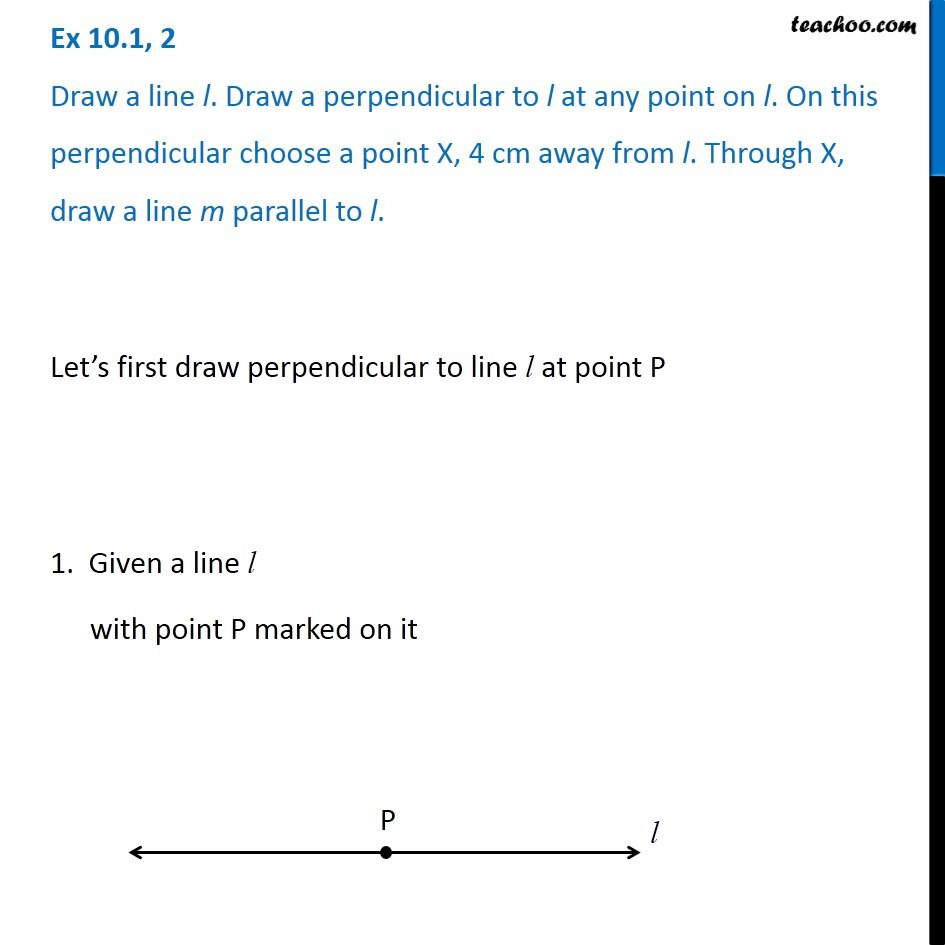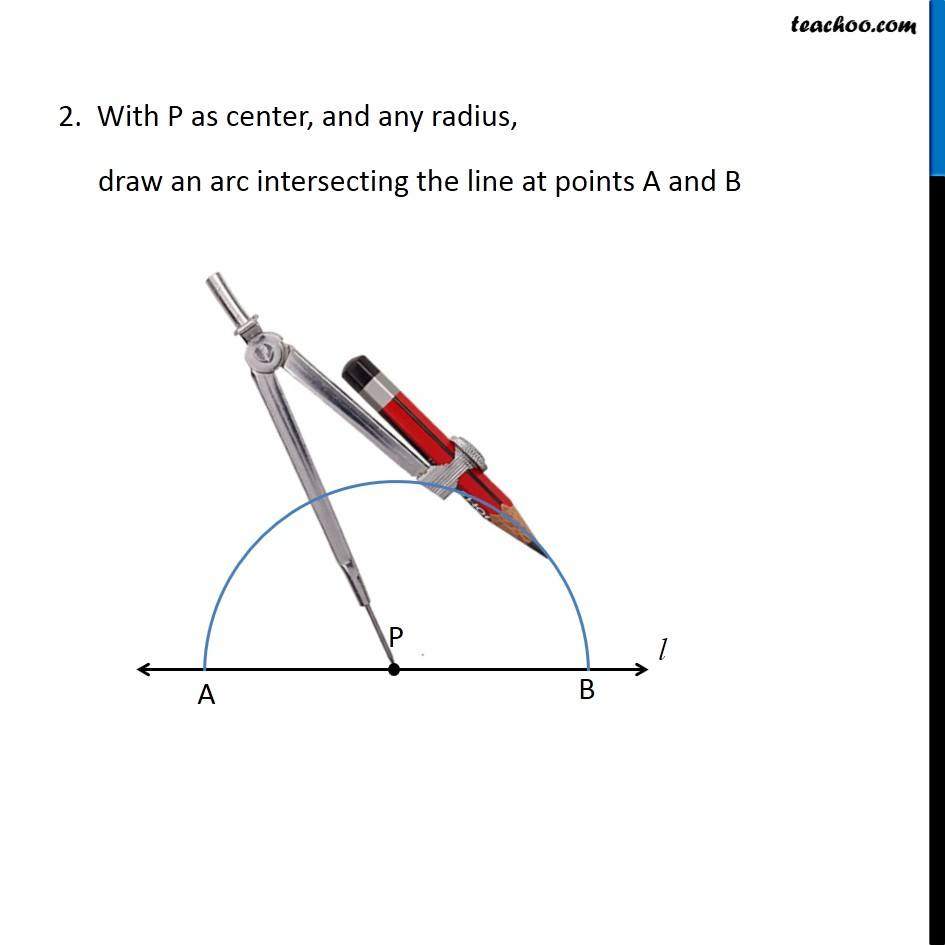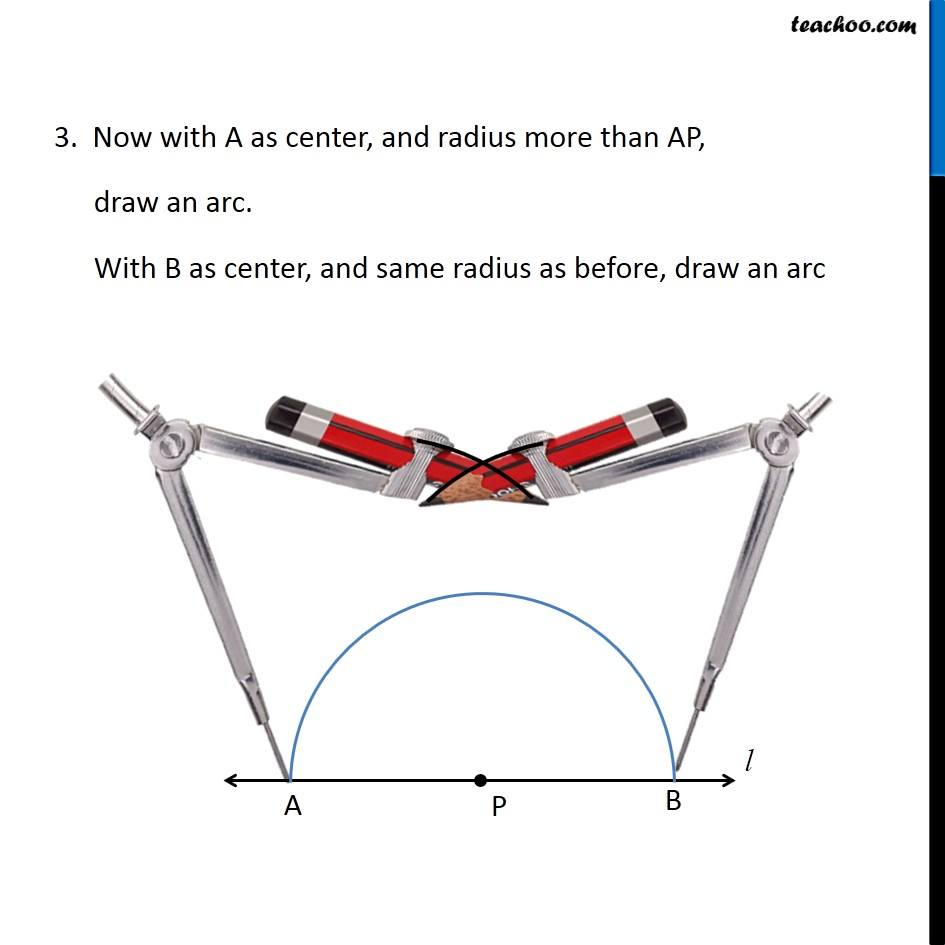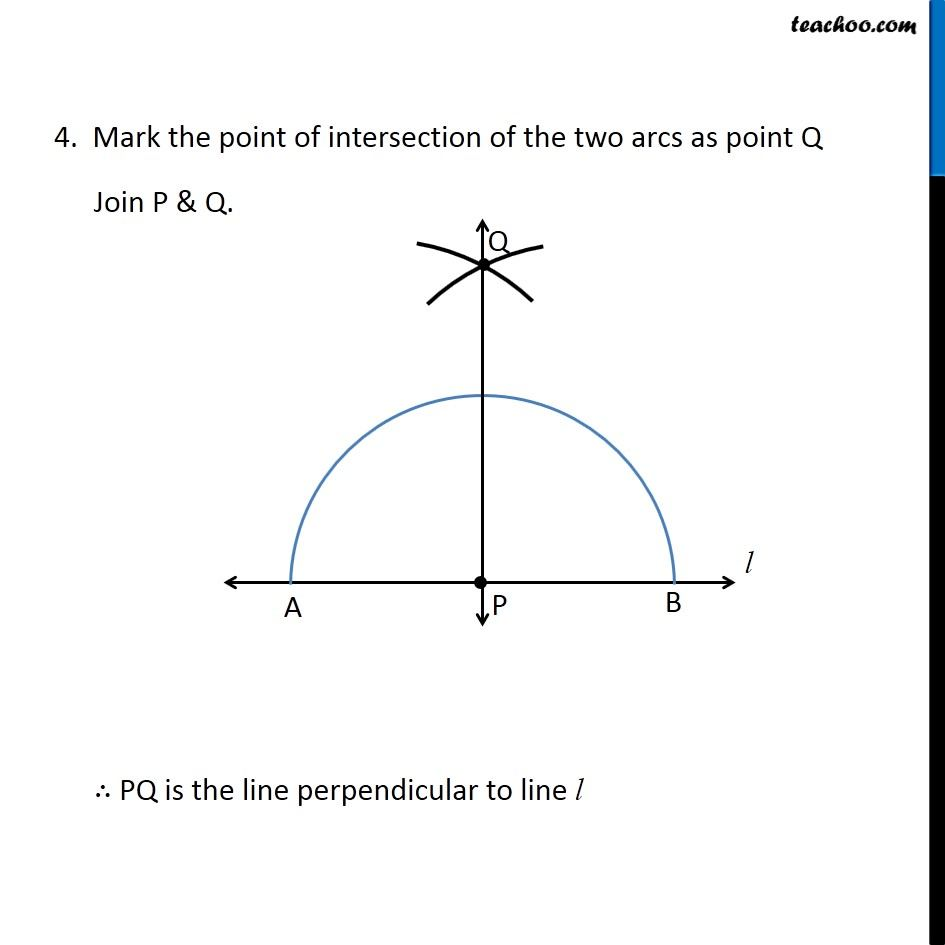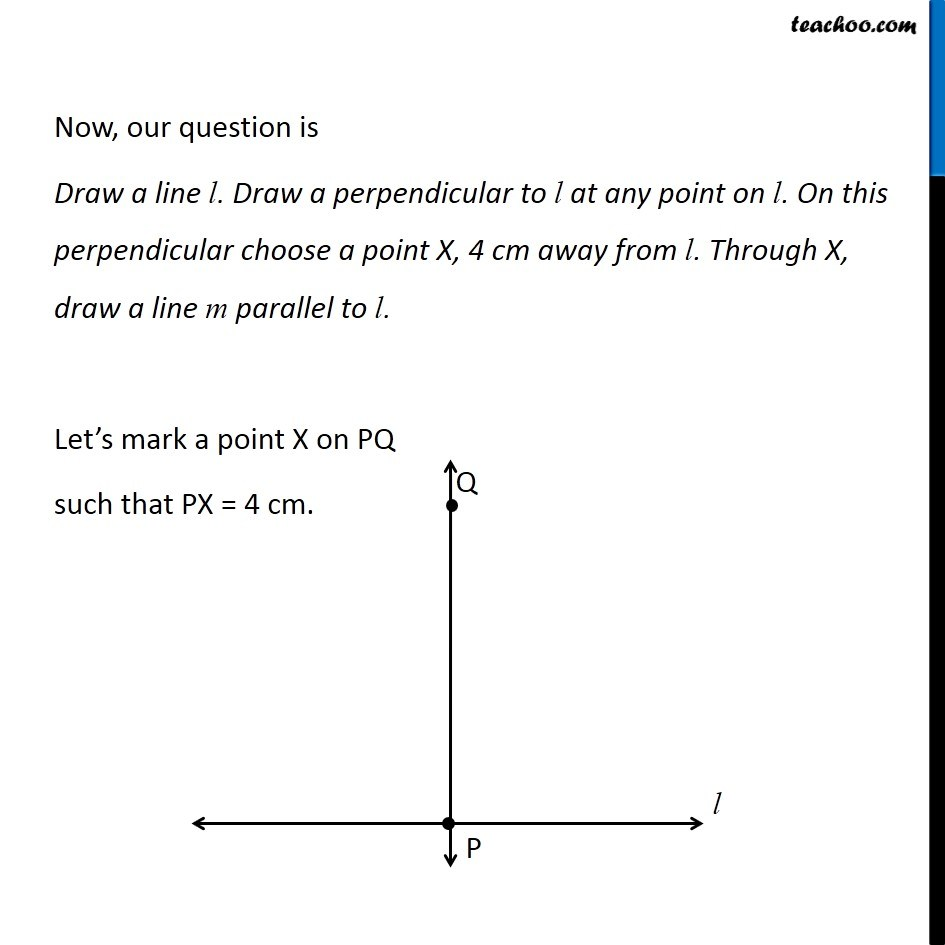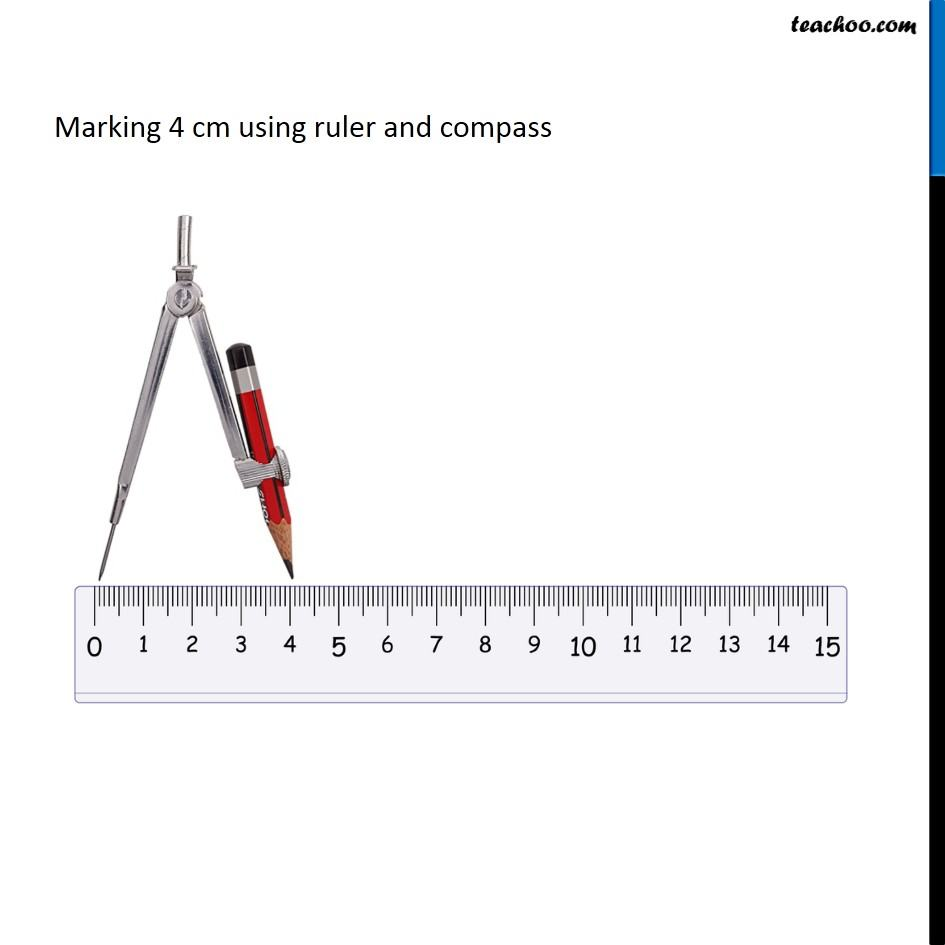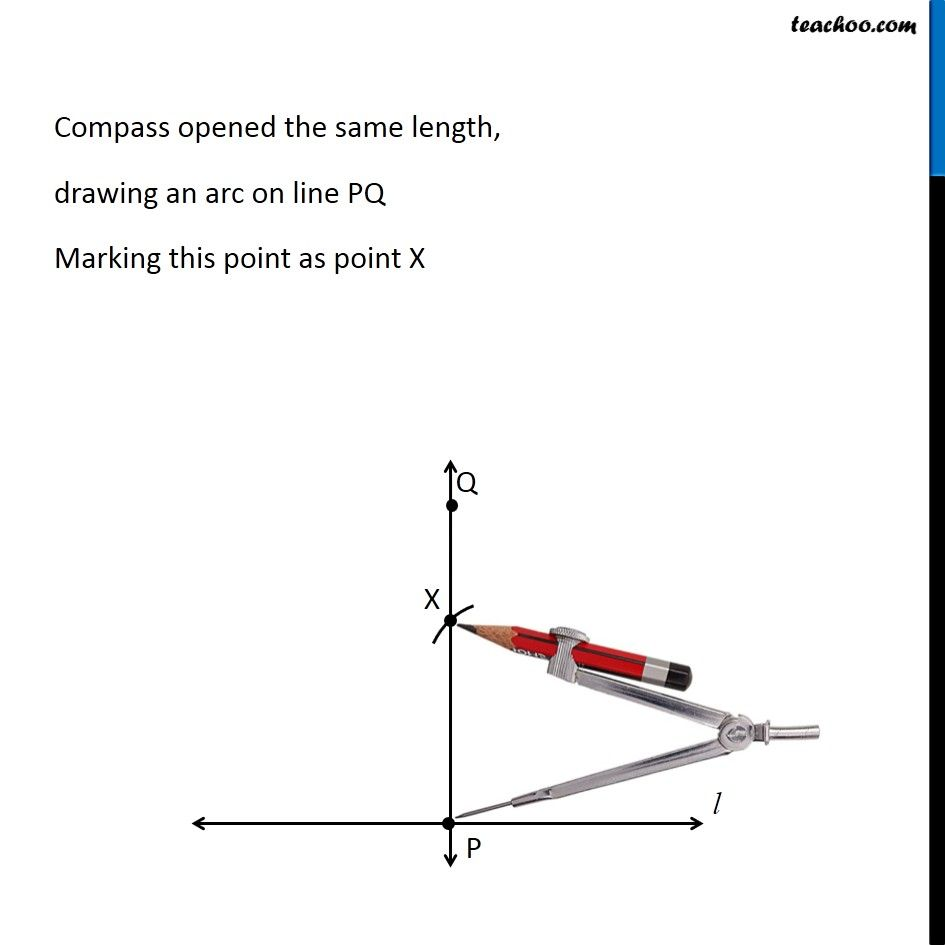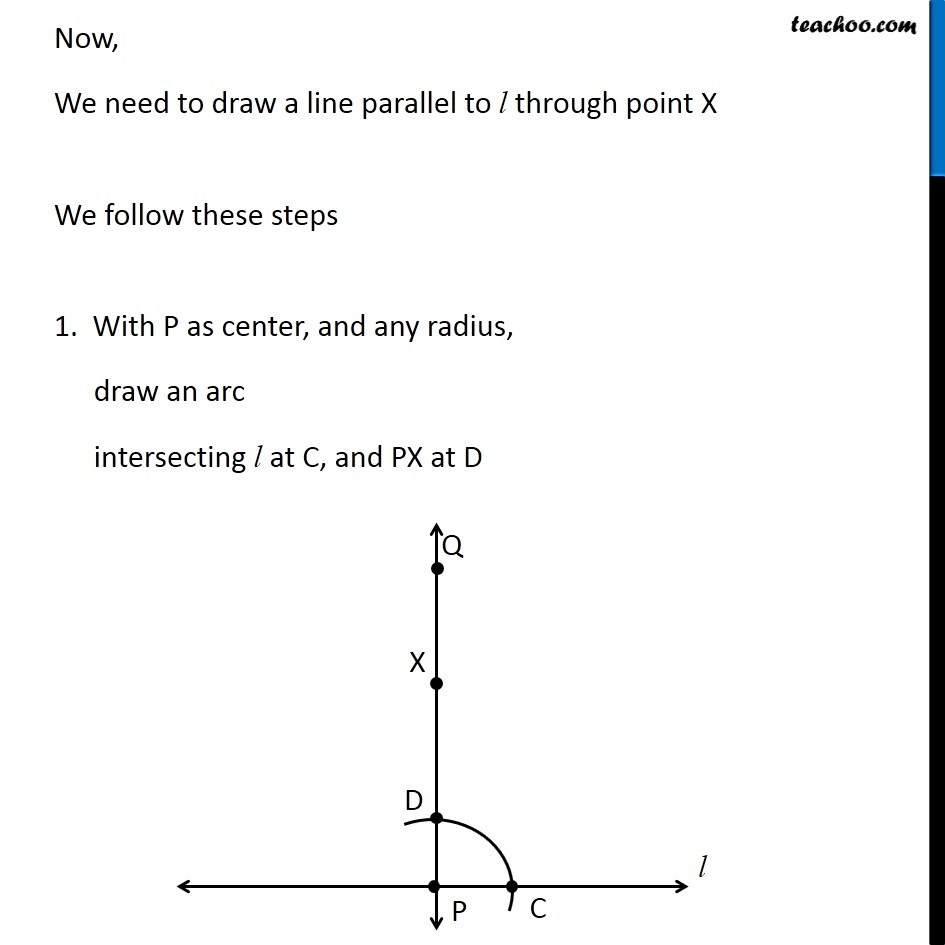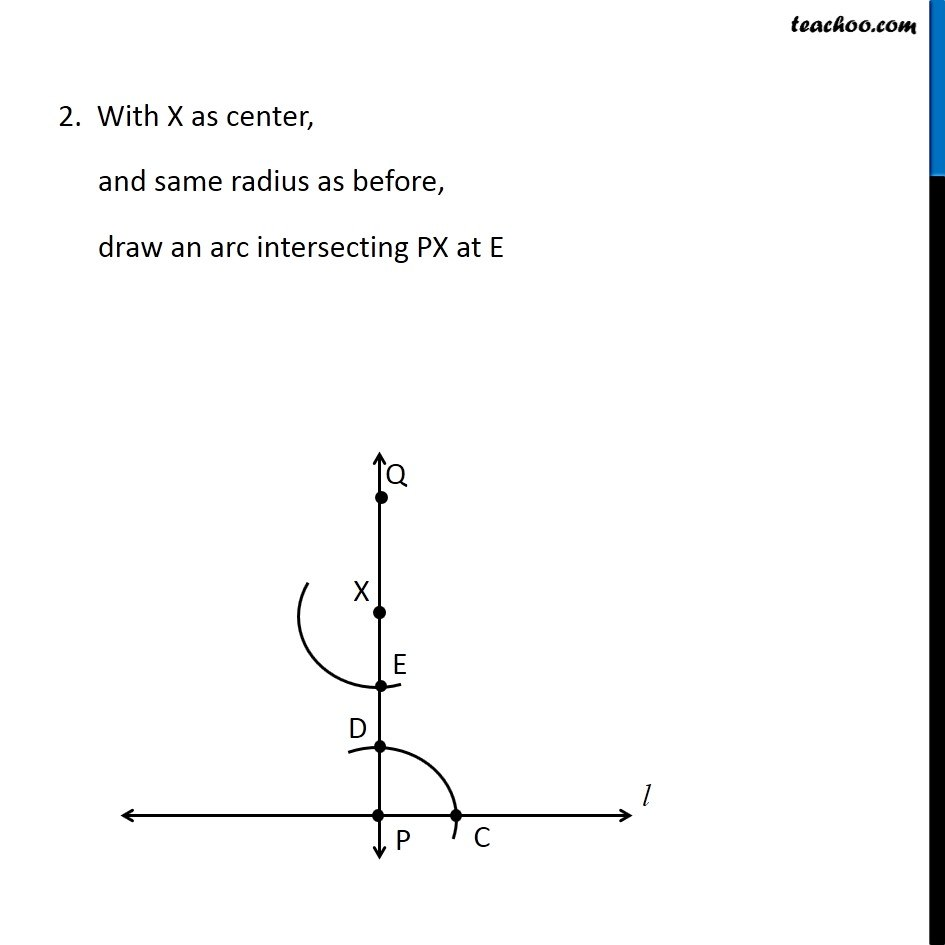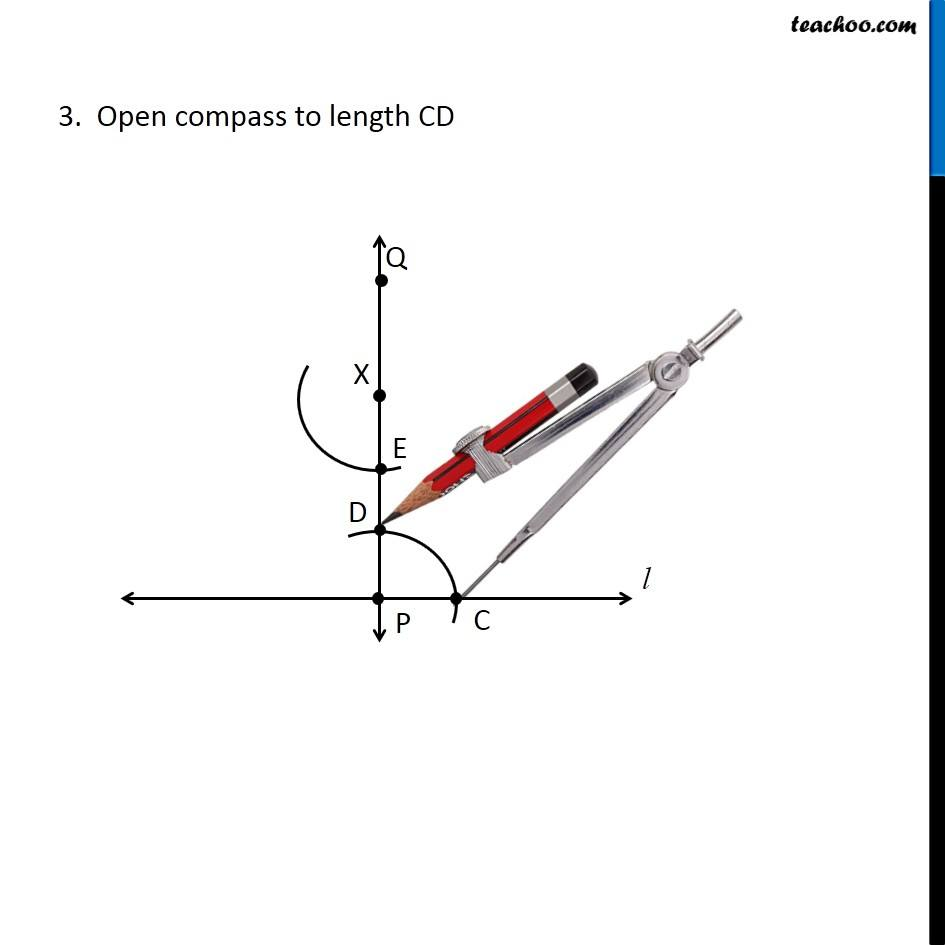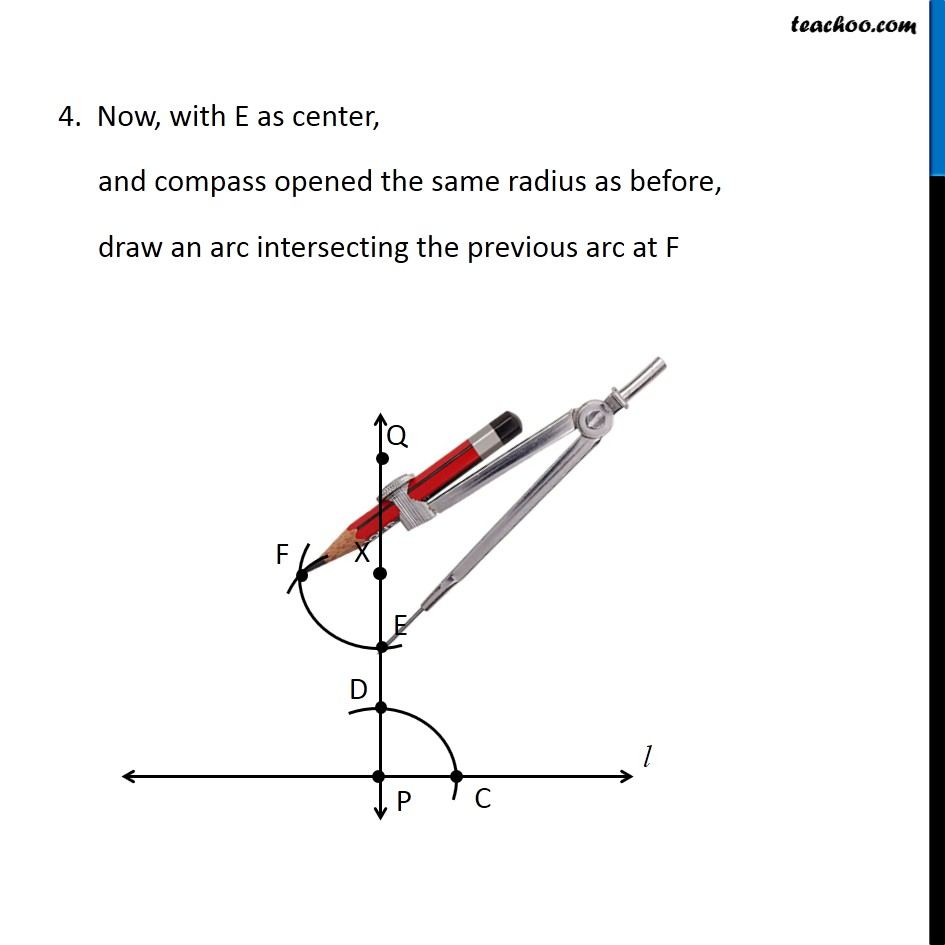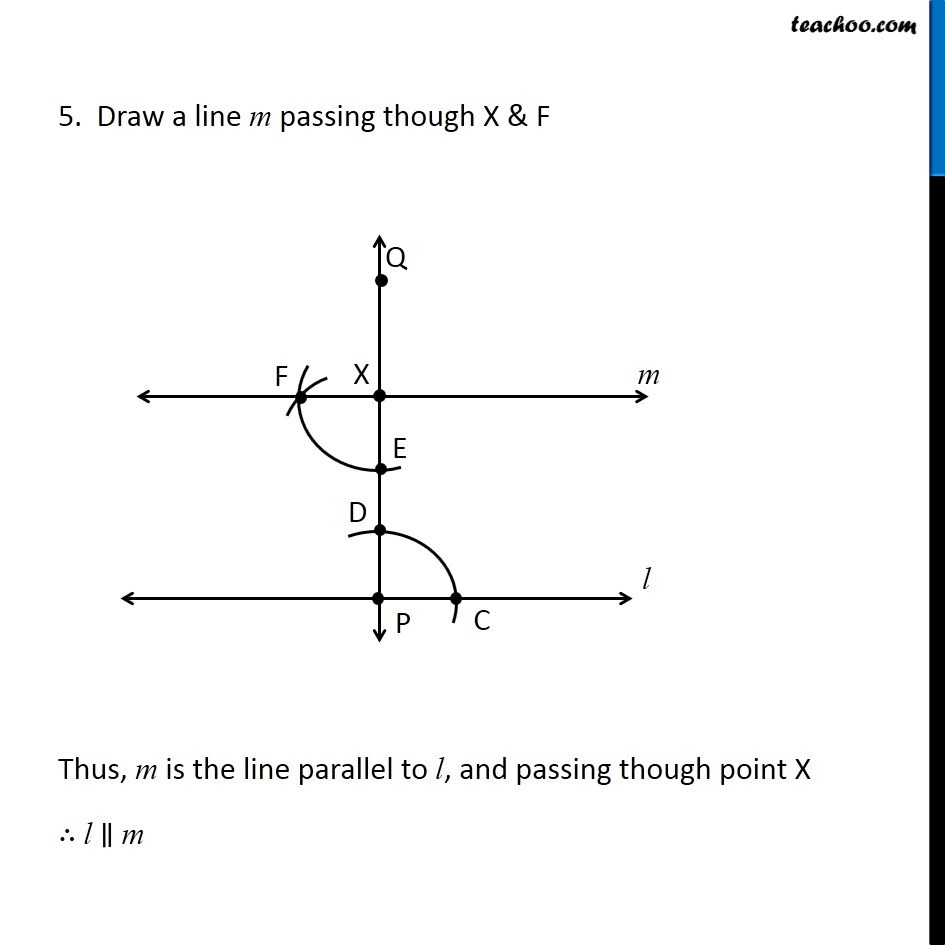Learn in your speed, with individual attention - Teachoo Maths 1-on-1 Class

### Transcript

Question 2 Draw a line l. Draw a perpendicular to l at any point on l. On this perpendicular choose a point X, 4 cm away from l. Through X, draw a line m parallel to l. Let’s first draw perpendicular to line l at point P 1. Given a line l with point P marked on it Let’s first draw perpendicular to line l at point P 1. Given a line l with point P marked on it 2. With P as center, and any radius, draw an arc intersecting the line at points A and B 3. Now with A as center, and radius more than AP, draw an arc. With B as center, and same radius as before, draw an arc 4. Mark the point of intersection of the two arcs as point Q Join P & Q. ∴ PQ is the line perpendicular to line l Now, our question is Draw a line l. Draw a perpendicular to l at any point on l. On this perpendicular choose a point X, 4 cm away from l. Through X, draw a line m parallel to l. Let’s mark a point X on PQ such that PX = 4 cm. Marking 4 cm using ruler and compass Compass opened the same length, drawing an arc on line PQ Marking this point as point X Compass opened the same length, drawing an arc on line PQ Marking this point as point X 2. With X as center, and same radius as before, draw an arc intersecting PX at E 3. Open compass to length CD 4. Now, with E as center, and compass opened the same radius as before, draw an arc intersecting the previous arc at F 5. Draw a line m passing though X & F Thus, m is the line parallel to l, and passing though point X ∴ l ∥ m